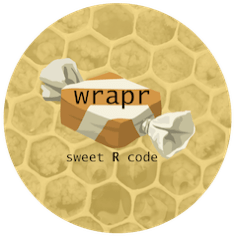# wrapr: R Code Sweeteners

`wrapr` is an R package that supplies powerful tools for writing and debugging R code.Primary `wrapr` services include:

• `let()`
• `%.>%` (dot arrow pipe)
• `:=` (named map builder)
• `λ()` (anonymous function builder)
• `DebugFnW()`

## `let()`

`let()` allows execution of arbitrary code with substituted variable names (note this is subtly different than binding values for names as with `base::substitute()` or `base::with()`).

The function is simple and powerful. It treats strings as variable names and re-writes expressions as if you had used the denoted variables. For example the following block of code is equivalent to having written "`a + a`".

``````library("wrapr")

a <- 7

let(
c(VAR = 'a'),

VAR + VAR
)
#   14``````

This is useful in re-adapting non-standard evaluation interfaces (NSE interfaces) so one can script or program over them.

We are trying to make `let()` self teaching and self documenting (to the extent that makes sense). For example try the arguments "`eval=FALSE`" prevent execution and see what would have been executed, or `debug=TRUE` to have the replaced code printed in addition to being executed:

``````let(
c(VAR = 'a'),
eval = FALSE,
{
VAR + VAR
}
)
#  {
#      a + a
#  }

let(
c(VAR = 'a'),
debugPrint = TRUE,
{
VAR + VAR
}
)
#  {
#      a + a
#  }
#   14``````

Please see `vignette('let', package='wrapr')` for more examples. For working with `dplyr` 0.7.* we suggest also taking a look at an alternate approach called `seplyr`.

## `%.>%` (dot arrow pipe)

`%.>%` dot arrow pipe is a strict pipe with intended semantics:

"`a %.>% b`" is to be treated as if the user had written "`{ . <- a; b };`" with "`%.>%`" being treated as left-associative.

That is: `%.>%` does not alter any function arguments that are not explicitly named. It is not defined as `a %.% b ~ b(a)` (roughly `dplyr`‘s original pipe) or as the large set of differing cases constituting `magrittr::%>%`. `%.>%` is designed to be explicit and simple.

The effect looks is show below.

The following two expressions should be equivalent:

``````cos(exp(sin(4)))
#   0.8919465

4 %.>% sin(.) %.>% exp(.) %.>% cos(.)
#   0.8919465``````

The notation is quite powerful as it treats pipe stages as expression parameterized over the variable "`.`". This means you do not need to introduce functions to express stages. The following is a valid dot-pipe:

``````1:4 %.>% .^2
#    1  4  9 16``````

The notation is also very regular in that expressions have the same iterpretation be then surrounded by parenthesis, braces, or as-is:

``````1:4 %.>% { .^2 }
#    1  4  9 16

1:4 %.>% ( .^2 )
#    1  4  9 16``````

Regularity can be a big advantage in teaching and comprehension. Please see "In Praise of Syntactic Sugar" for more details.

## `:=`

`:=` is the "named map builder". It allows code such as the following:

``````'a' := 'x'
#    a
#  "x"``````

The important property of named map builder is it accepts values on the left-hand side allowing the following:

``````name <- 'variableNameFromElsewhere'
name := 'newBinding'
#  variableNameFromElsewhere
#               "newBinding"``````

A nice property is `:=` commutes (in the sense of algebra or category theory) with `R`‘s concatenation function `c()`. That is the following two statements are equivalent:

``````c('a', 'b') := c('x', 'y')
#    a   b
#  "x" "y"

c('a' := 'x', 'b' := 'y')
#    a   b
#  "x" "y"``````

## `λ()`

`λ()` is a concise abstract function creator. It is a placeholder that allows the use of the λ-character for very concise function abstraction.

Example:

``````# Make sure lambda function builder is in our enironment.
wrapr::defineLambda()

# square numbers 1 through 4
sapply(1:4, λ(x, x^2))
#    1  4  9 16``````

## `DebugFnW()`

`DebugFnW()` wraps a function for debugging. If the function throws an exception the execution context (function arguments, function name, and more) is captured and stored for the user. The function call can then be reconstituted, inspected and even re-run with a step-debugger. Please see our free debugging video series and `vignette('DebugFnW', package='wrapr')` for examples.

Categories: Programming Statistics### jmount

Data Scientist and trainer at Win Vector LLC. One of the authors of Practical Data Science with R.